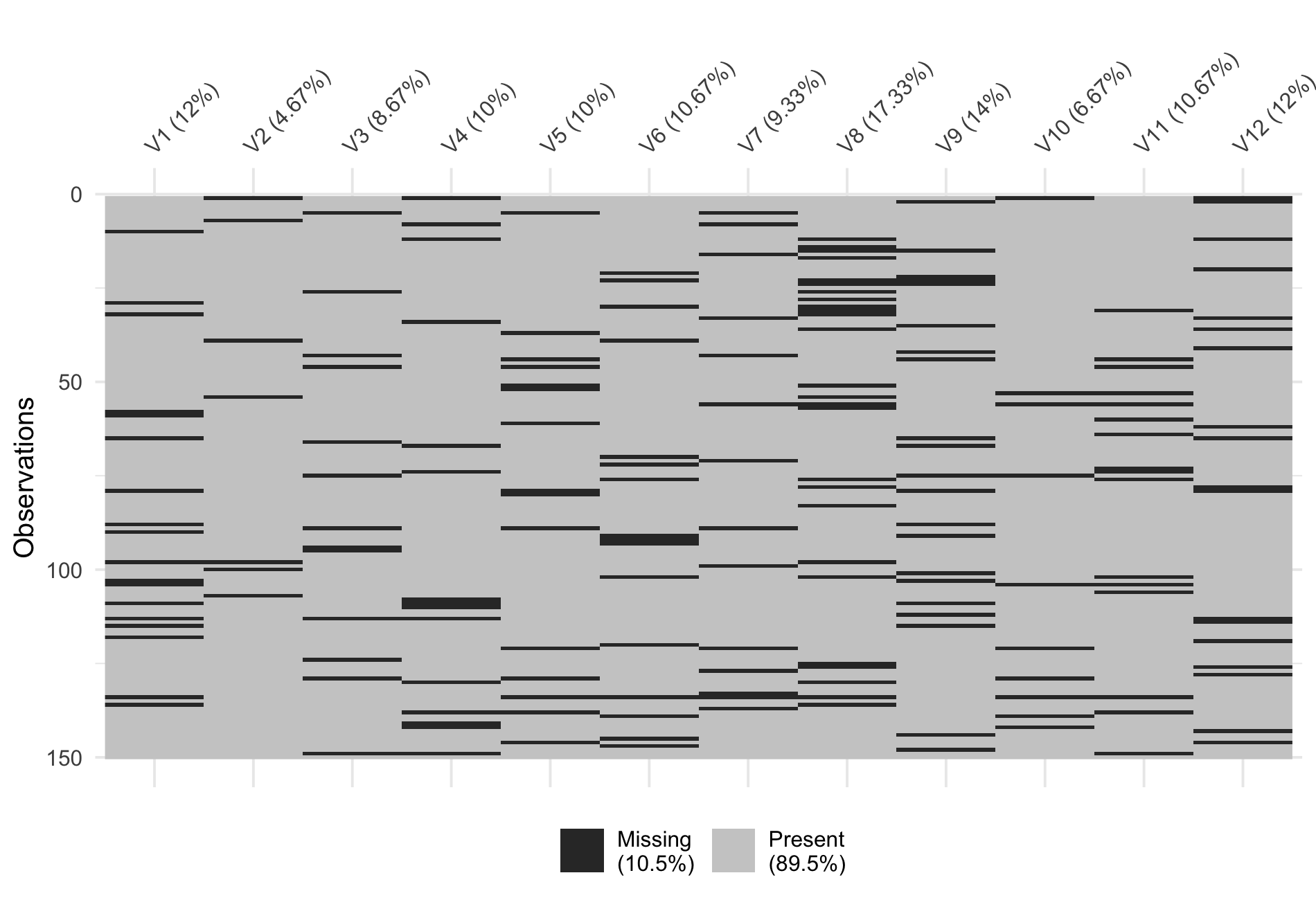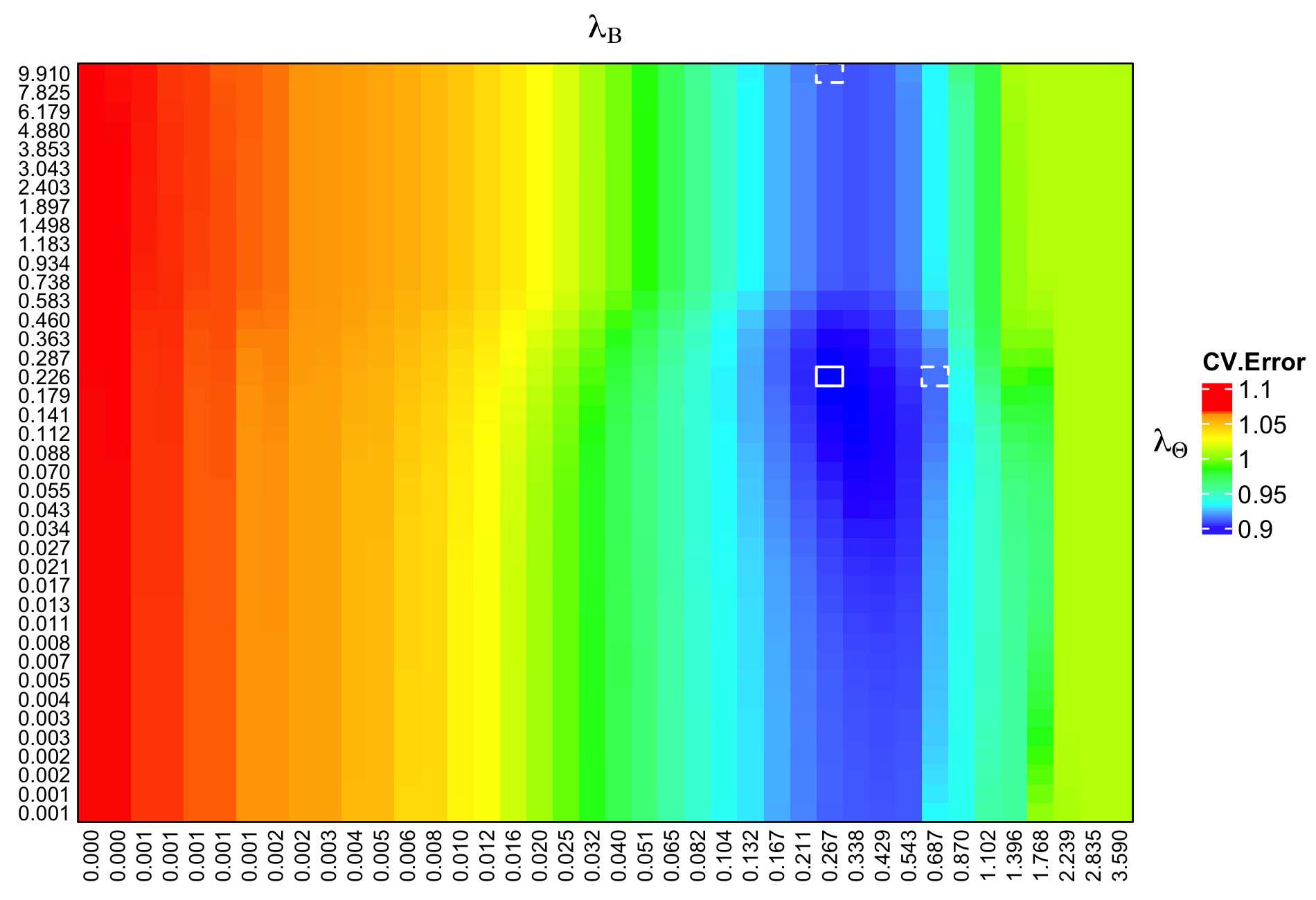# 1 Introduction

missoNet is a package that fits penalized multi-task regression – that is, with multiple correlated tasks or response variables – to simultaneously estimate the coefficients of a set of predictor variables for all tasks and the conditional response network structure given all predictors, via penalized maximum likelihood in an undirected conditional Gaussian graphical model. In contrast to most penalized multi-task regression (conditional graphical lasso) methods, missoNet has the capability of obtaining estimates even when the response data is corrupted by missing values. The method automatically enjoys the theoretical and computational benefits of convexity, and returns solutions that are comparable/close to the estimates without any missing values.

missoNet assumes the model $\mathbf{Y} = \mathbf{X}\mathbf{B}^* + \mathbf{E},\ \ \mathbf{E}_{i\cdot} \stackrel{iid}{\sim} \mathcal{MVN}(0_q,(\mathbf{\Theta}^*)^{-1})\ \ \forall i = 1,...,n,$ where $$\mathbf{Y} \in \mathbb{R}^{n\times q}$$ and $$\mathbf{X} \in \mathbb{R}^{n\times p}$$ represent the centered response data matrix and predictor data matrix, respectively (thus the intercepts are omitted). $$\mathbf{E}_{i\cdot} \in \mathbb{R}^{q}$$ is the $$i$$th row ($$i$$th sample) of the error matrix and is drawn from a multivariate Gaussian distribution. $$n, p, q$$ denote the sample size, the number of predictors and the number of responses, respectively. The regression coefficient matrix $$\mathbf{B}^* \in \mathbb{R}^{p\times q}$$ and the precision (inverse covariance) matrix $$\mathbf{\Theta}^* \in \mathbb{R}^{q\times q}$$ are parameters to be estimated in this model. Note that the entries of $$\mathbf{\Theta}^*$$ have a one-to-one correspondence with partial correlations. That is, the random variables $$Y_j$$ and $$Y_k$$ are conditionally independent (i.e. $$\mathbf{\Theta}^*_{jk} = 0$$) given $$X$$ and all other remaining variables in $$Y$$ iff the corresponding partial correlation coefficient is zero.

To estimate the parameters, missoNet solves a penalized MLE problem: $(\hat{\mathbf{\Theta}},\hat{\mathbf{B}}) = \underset{\mathbf{\Theta} \succeq 0,\ \mathbf{B}}{\text{argmin}}\ \mathrm{tr}\left[\frac{1}{n}(\mathbf{Y} - \mathbf{XB})^\top(\mathbf{Y} - \mathbf{XB}) \mathbf{\Theta}\right] - \mathrm{log}|\mathbf{\Theta}| + \lambda_{\Theta}(\|\mathbf{\Theta}\|_{1,\mathrm{off}} + 1_{n\leq \mathrm{max}(p,q)} \|\mathbf{\Theta}\|_{1,\mathrm{diag}}) + \lambda_{B}\|\mathbf{B}\|_1.$ $$\mathbf{B}^*$$ that mapping predictors to responses and $$\mathbf{\Theta}^*$$ that revealing the responses’ conditional dependencies are estimated for the lasso penalties – $$\lambda_B$$ and $$\lambda_\Theta$$, over a grid of values (on the log scale) covering the entire range of possible solutions. To learn more about sparse multi-task regression with inverse covariance estimation using datasets without missing values, see MRCE.

However, missingness in real data is inevitable. Most penalized multi-task regression / conditional graphical lasso methods either assume the data is fully-observed (eg. MRCE), or deal with missing data through a likelihood-based method such as the EM algorithm (e.g, cglasso). An important challenge in this context is the possible non-convexity of the associated optimization problem when there is missing data, as well as the high computational cost from iteratively updating the estimations for parameters.

missoNet aims to handle a specific class of datasets where the response matrix $$\mathbf{Y}$$ contains data which is missing at random (MAR). To use missoNet, users do not need to possess additional knowledge for pre-processing missing data (e.g. imputation) nor for selection of regularization parameters ($$\lambda_B$$ and $$\lambda_\Theta$$), the method provides a unified framework for automatically solving a convex modification of the multi-task learning problem defined above, using corrupted datasets.

The package provides an integrated set of core routines for data simulation, model fitting and cross-validation, visualization of results, as well as predictions in new data. The function arguments are in the same style as those of glmnet, making it easy for experienced users to get started.

# 2 Installation

To install the package missoNet from CRAN, type the following command in the R console:

install.packages("missoNet")

Or install the development version of missoNet from GitHub:

if(!require("devtools")) {
install.packages("devtools")
}
devtools::install_github("yixiao-zeng/missoNet")

# 3 Quick Start

The purpose of this section is to give users a general overview of the functions and their usages. We will briefly go over the main functions, basic operations and outputs.

First, we load the missoNet package:

library(missoNet)

We will use a synthetic dataset for illustration. To generate a set of data with missing response values, we can simply call the built-in function generateData:

## Specify a random seed for reproducibility.
## The overall missing rate in the response matrix is around 10%.
## The missing mechanism can also be "MCAR" or "MNAR".
sim.dat <- generateData(n = 150, p = 15, q = 12, rho = 0.1, missing.type = "MAR", with.seed = 1512)

tr <- 1:120  # training set indices
tst <- 121:150  # test set indices

This command returns an object containing all necessary components for analysis including:

• 'X', 'Y': a predictor matrix $$\mathbf{X} \in \mathbb{R}^{n\times p}$$ and a complete (clean) response matrix $$\mathbf{Y} \in \mathbb{R}^{n\times q}$$, in which rows correspond to samples and columns correspond to variables. The rows of $$\mathbf{Y}$$ is sampled from $$\mathcal{MVN}(X\mathbf{B}^*,(\mathbf{\Theta}^*)^{-1})$$.

• 'Beta', 'Theta': the true parameters $$\mathbf{B}^* \in \mathbb{R}^{p\times q}$$ and $$\mathbf{\Theta}^* \in \mathbb{R}^{q\times q}$$ for the generative model that will be estimated later (see the later section for more details on their structures and sparsity).

• 'Z': a corrupted response matrix $$\mathbf{Z} \in \mathbb{R}^{n\times q}$$ having elements $$\mathbf{Z}_{ij}$$ ($$\forall\ i=1,...,n,\ \ j=1,...,q$$) which is either NA or equal to $$\mathbf{Y}_{ij}$$.

The probabilities of missingness for the response variables and the missing mechanism are specified by 'rho' and 'missing.type', respectively. 'rho' can be a scalar or a vector of length $$q$$. In the code above, 'rho' = 0.1 indicates an overall missing rate of around 10%. In addition to "MAR", the 'missing.type' can also be "MCAR" or "MNAR", see the later section for more details on how missing values are generated under different mechanisms.

NOTE 1: the program only accepts missing values that are coded as either NAs or NaNs.

NOTE 2: although the program will provide estimates even when $$n \leq \text{max}(p,q)$$, convergence is likely to be rather slow, and the variance of estimation is likely to be excessively large. Therefore, we suggest that both the predictor columns and the response columns be reduced (filtered) if possible, prior to fitting any models. For example in genomics, where $$\mathbf{X}$$ can very wide (i.e. large $$p$$), we often filter features based on variance or coefficient of variation.

We can easily visualize how missing data is distributed in the corrupted response matrix $$\mathbf{Z}$$ using package visdat:

Z <- sim.dat$Z # corrupted response matrix visdat::vis_miss(as.data.frame(Z))A single model can be fitted using the most basic call to missoNet: ## Training set X.tr <- sim.dat$X[tr, ]  # predictor matrix
Z.tr <- sim.dat$Z[tr, ] # corrupted response matrix ## Using the training set to fit the model. ## 'lambda.Beta' and 'lambda.Theta' are arbitrarily set to 0.1. ## 'verbose' = 0 suppresses printing of messages. fit <- missoNet(X = X.tr, Y = Z.tr, lambda.Beta = 0.1, lambda.Theta = 0.1, verbose = 0) However, missoNet should be more commonly used with a grid of values for $$\lambda_B$$ and $$\lambda_\Theta$$. The two penalty vectors must have the same length, and the missoNet function will be called with each consecutive pair of values – i.e. with the first elements of the two vectors, then with the second elements, etc. lambda.Beta.vec <- 10^(seq(from = 0, to = -1, length.out = 10)) # 10 values on the log scale, from 1 to 0.1 lambda.Theta.vec <- rep(0.1, 10) # for each value of 'lambda.Beta', 'lambda.Theta' remains constant at 0.1 fit_list <- missoNet(X = X.tr, Y = Z.tr, lambda.Beta = lambda.Beta.vec, lambda.Theta = lambda.Theta.vec, verbose = 0) The command above returns a sequence of models for users to choose from. In many cases, users may prefer that the software selects the best fit among them. Cross-validation is perhaps the simplest and most widely used method for this task. cv.missoNet is the main function to do cross-validation, along with supporting methods such as plot and predict. Here we use cv.missoNet to perform a five-fold cross-validation, samples will be permuted before splitting into multiple folds. For reproducibility, we assign a random seed to the permutation. ## If 'permute' = FALSE, the samples will be split into k-folds in their original orders, ## i.e. the first (n/'kfold') samples will be assigned to the first fold an so on. cvfit <- cv.missoNet(X = X.tr, Y = Z.tr, kfold = 5, lambda.Beta = lambda.Beta.vec, lambda.Theta = lambda.Theta.vec, permute = TRUE, with.seed = 433, verbose = 0) #> Warning in boundaryCheck(lambda.Theta = lambda.Theta, lambda.Beta = lambda.Beta, : #> The optimal lambda.Theta is close to the upper boundary of the search range, #> try to provide a new sequence covering larger values for the following argument: #> #> 1. lambda.Theta The program has warned us that the range of $$\lambda_\Theta$$ is inappropriate so that we need to supply a sequence of $$\lambda_\Theta$$ covering larger values. However, picking a suitable range for such hyperparameters may require experience or a series of trials and errors. Therefore, cv.missoNet provides a smarter way to solve this problem. Let’s fit the cross-validation model again, this time running all folds in parallel on two CPU cores. The parallelization of missoNet models relies on package parallel, so make sure the parallel clusters have been registered beforehand. ## 'fit.1se = TRUE' tells the program to make additional estimations of the ## parameters at the largest value of 'lambda.Beta' / 'lambda.Theta' that gives ## the most regularized model such that the cross-validated error is within one ## standard error of the minimum. cl <- parallel::makeCluster(min(parallel::detectCores()-1, 2)) cvfit <- cv.missoNet(X = X.tr, Y = Z.tr, kfold = 5, fit.1se = TRUE, parallel = TRUE, cl = cl, permute = TRUE, with.seed = 433, verbose = 1) parallel::stopCluster(cl) Note that we have not explicitly specified the regularization parameters $$\lambda_B$$ and $$\lambda_\Theta$$ in the command above. In this case, a grid of $$\lambda_B$$ and $$\lambda_\Theta$$ values in a (hopefully) reasonable range will be computed and used by the program. Users can even provide just one of $$\lambda_B$$ and $$\lambda_\Theta$$ vectors such that the program will compute the other. Generally, it is difficult at the beginning to choose suitable $$\lambda$$ sequences, so we suggest users start analysis using cv.missoNet, and let the program compute the appropriate $$\lambda_B$$ and $$\lambda_\Theta$$ values itself, and then automatically pick the optimal combination of them at which the minimum cross-validated error is achieved. cv.missoNet returns a 'cv.missoNet' object, a list with all the ingredients of the cross-validated fit. We can execute the plot method ## The values of mean cross-validated errors along with upper and lower standard deviation bounds ## can be accessed via 'cvfit$cvm', 'cvfit$cvup' and 'cvfit$cvlo', respectively.

plot(cvfit)to visualize the mean cross-validated error in a heatmap style. The white solid box marks out the position of the minimum mean cross-validated error with corresponding $$\lambda_B$$ and $$\lambda_\Theta$$ ("lambda.min" := $$({\lambda_B}_\text{min}, {\lambda_\Theta}_\text{min})$$), and the white dashed boxes indicate the largest $$\lambda_B$$ and $$\lambda_\Theta$$ at which the mean cross-validated error is within one standard error of the minimum, by fixing the other one at "lambda.min" (i.e. "lambda.1se.Beta" := $$({\lambda_B}_\text{1se}, {\lambda_\Theta}_\text{min})$$ and "lambda.1se.Theta" := $$({\lambda_B}_\text{min}, {\lambda_\Theta}_\text{1se})$$). We often use this “one-standard-error” rule when selecting the most parsimonious model; this acknowledges the fact that the cross-validation surface is estimated with error, so error on the side of parsimony is preferable.

We can also plot the errors in a 3D scatter plot (without surfaces):

plot(cvfit, type = "cv.scatter", plt.surf = FALSE)